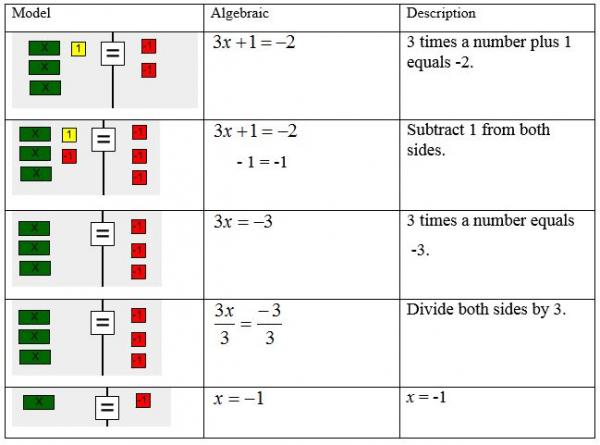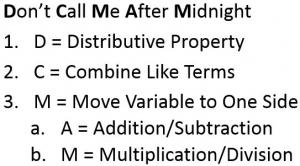# Let's Get Started

TEKS Standards and Student Expectations

A(5) The student applies the mathematical process standards to solve, with and without technology, linear equations and evaluate the reasonableness of their solutions. The student is expected to:

A(5)(A) solve linear equations in one variable, including those for which the application of the distributive property is necessary and for which variables are included on both sides

A(5)(B) solve linear inequalities in one variable, including those for which the application of the distributive property is necessary and for which variables are included on both sides

Resource Objective(s)

The student will use a variety of methods to solve equations and inequalities with variables on both sides.

Essential Questions

How can algebra tiles be used to solve equations and inequalities?

What are the steps used to solve equations and inequalities algebraically?

How can an equation or inequality be written in standard form?

Vocabulary

# Solving One-Variable Equations: Part 1

To solve equations, you must isolate the variable. Zero pairs and inverse operations can be used to eliminate constants in an equation.

The example below shows how to solve equations using models and algebraic steps.For more practice solving two-step equations, click the image below to open the interactive tool. Follow the “Feedback” directions beneath the work mat to build the model with algebra tiles and solve the equation.

# Solving One-Variable Equations: Part 2

Some equations can be more complicated and require multiple steps in order to solve them. The acronym "Don't Call Me After Midnight" can be used to help you remember the steps to solving equations.Example 1

Solve for x in the following equation: 2x - 4 = x + 5.

Step 1: Move all variables to one side of the equation by subtracting x from both sides.

2x - 4 = x + 5
-x         -x
x - 4 = 5

Step 2: Add 4 to both sides of the equation.

- 4 = 5
+4  +4
x = 9

Example 2

Solve for x in the following equation: 3x + 15 - 9 = 2(x +2).

Step 1: Distribute 2 to the (x + 2) by multiplying by 2.

3x + 15 - 9 = 2(x + 2)
3x + 15 - 9 = 2x + 4

Step 2: Combine like terms by subtracting 9 from 15 (15 - 9 = 6).

3x + 6 = 2x + 4

Step 3: Move all variables to one side of the equation by subtracting 2x from both sides.

3x + 6 = 2x + 4
-2x        -2x
x + 6 = 4

Step 4: Subtract 6 from both sides.

x + 6 = 4
-6   -6
x = -2

# Transforming Linear Equations

We're going to learn how to transform an equation or inequality into an equivalent equation or inequality. This involves rearranging the values of the inequality or equation by using inverse operations. Let's investigate different ways to transform linear equations from one representation to another.

The most common transformation of a linear equation that you will need to know is how to take an equation in standard form (Ax + By = C) and rewrite it in slope-intercept form (y = mx + b), or vice versa. This transformation is important because the two different forms reveal different types of information quickly.

The table below shows the important information in each form.

 Form of Linear Equation Important Information Where You Will See This Form Slope-Intercept Form y = mx + b The slope is m. The y-coordinate of the y-intercept is b. Problems that include a starting point (b) and a rate of change. Standard Form Ax + By = C The x-coordinate of the x-intercept is C/A. The y-coordinate of the y-intercept is C/B. Problems that include a combination of multiples of x and y.

To transform from standard form to slope-intercept form usually requires two steps.

Step 1. Add or subtract the x term from both sides.

Step 2. Divide all terms by the coefficient of y.

Example 1

Transform the equation into slope-intercept form:

To transform from slope-intercept form to standard form usually requires no more than four steps.

Step 1. Add or subtract the x term from both sides.

Step 2. If the coefficient of x is negative (the A term), multiply all terms by -1.

Step 3. If there is a fraction, multiply all terms by the denominator to eliminate fractions.

Step 4. If there is a decimal, multiply all terms by a power of 10 to eliminate decimals.

Example 2

Transform the equation into standard form:

Check your understanding by completing the following prompts.

# Transforming Linear Inequalities

Linear inequalities can be transformed in a way that is similar to linear equalities. This first example shows how to transform an inequality.

Example 1

What is another way to write the following inequality?

3x + 2y ≥ 6

In order to write the inequality another way, we must make sure that the value of the solutions remains the same.

We can rewrite the inequality by solving for y, OR we can rewrite the inequality by solving for x. Let’s try both.When working with inequalities, it is important to remember that the inequality symbol must reverse (or flip) when you multiply or divide by a negative number.

Watch the following video on solving equations and inequalities and answer the prompts when they pop up. When you're done viewing, follow the steps below to convert a equation from standard form to slope-intercept form.